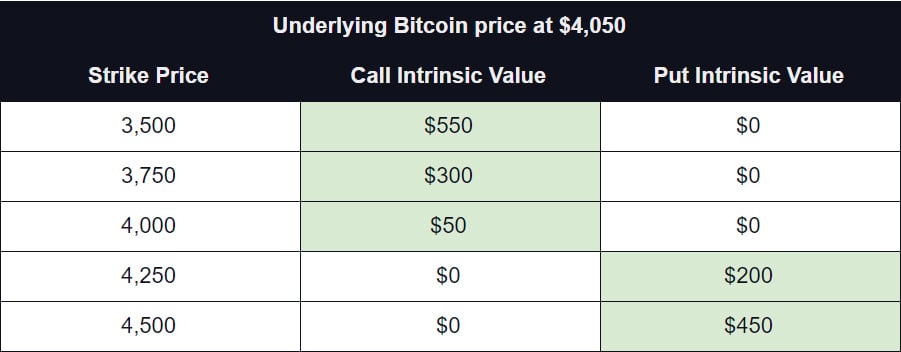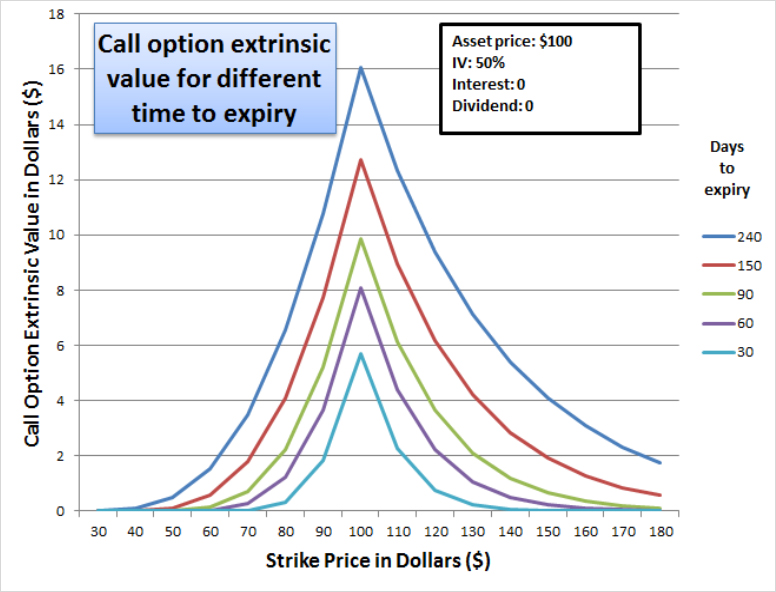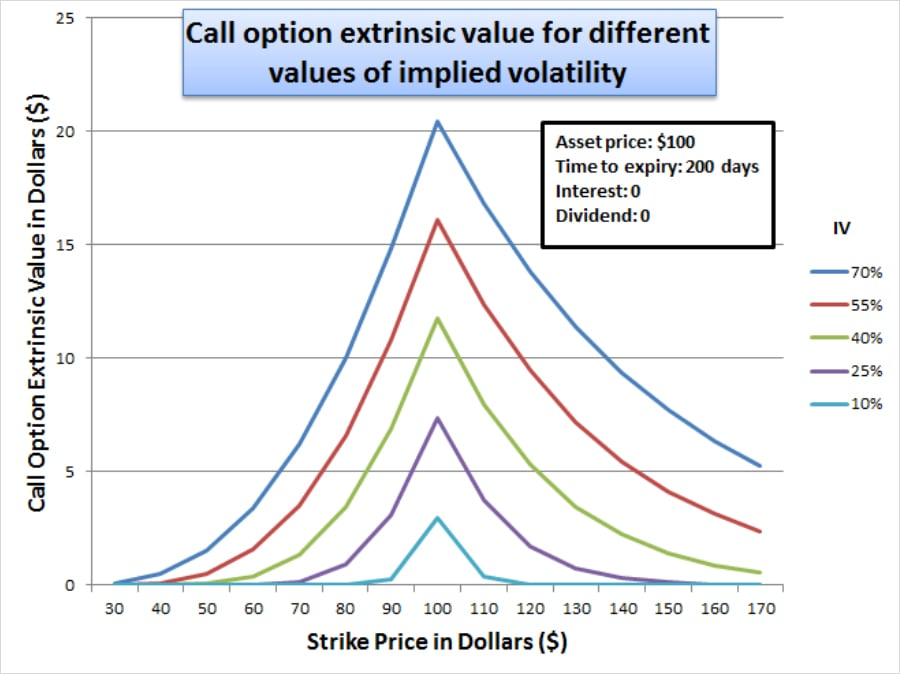An option value can be determined by its intrinsic value and its extrinsic value.

## Intrinsic value

The intrinsic value shows how far in the money the option is, or the difference between the option’s strike price and the current price of the underlying asset.

For example if you own a Bitcoin call option with a strike price of \$3,500 and the current price of BTC is \$4,050 then the intrinsic value of that option is \$4,050 – \$3,500 = \$550.

Options only have an intrinsic value when they are in the money, so if an option is out of the money, its intrinsic value is zero.

An option is in the money, when its intrinsic value is positive.

• Intrinsic value for a call option is calculated as:

Underlying Price – Strike Price

• Intrinsic value for a put option is calculated as:

Strike Price – Underlying Price

Here are some examples of the intrinsic value of options with various strike prices, given a BTC price of \$4,050.## Extrinsic value

An options extrinsic value is any extra value it has when intrinsic value is discluded from the option price.

• Extrinsic Value = Option Price – Intrinsic Value

For example in the table above, if the call option with a strike price of \$3,750 is priced at \$500, then its extrinsic value is \$200.

• Extrinsic Value = Option Price – Intrinsic Value = \$500 – \$300 = \$200

There are several factors that determine the extrinsic value. It is affected by the time until expiry, implied volatility, interest free rate, dividend rate and the distance between the strike price and underlying price. The most common option pricing model for European style options is the Black Scholes model, it includes all the above mentioned parameters, and allows the option contract price to be determined. This model is also used on Deribit.

On Deribit the interest free rate and dividend parameters are left at zero, as these parameters are not accounted for in crypto markets. Therefore, let’s look at an example of how time to expiry and implied volatility affect the extrinsic value of an options contract.

In the chart below you can see how different times to expiry will affect the extrinsic value of options at each strike price. This is a fictional asset with a current price of \$100 and a fixed implied volatility of 50%.All other things being equal, the more time an option has until expiration, the higher its extrinsic value. However, the progression is not linear. With 240 days to expiry the extrinsic value of the call option with \$100 strike is ~\$16, however it is not until 60 days to expiry that extrinsic value is halved to ~\$8. More on this in an upcoming lesson.

In the chart below you can see how changes in implied volatility will affect the extrinsic value of options at each strike price. Again this is a fictual asset with a current price of \$100 and 200 days to expiry.All other things being equal, the higher the IV, the higher the extrinsic value of the option. Altogether, the more time an option contract has until expiry and the more volatile the price of the underlying asset, the higher the option’s premium.

Option’s highest extrinsic value is always for at the money (Strike Price = Price of the underlying asset) options (\$100 in this case). This decreases as the price of the underlying asset moves in either direction away from the strike price.

Note: these charts only show the extrinsic value. The intrinsic component of the ITM options has been removed.

AUTHOR(S)CryptarbitrageMrJozza